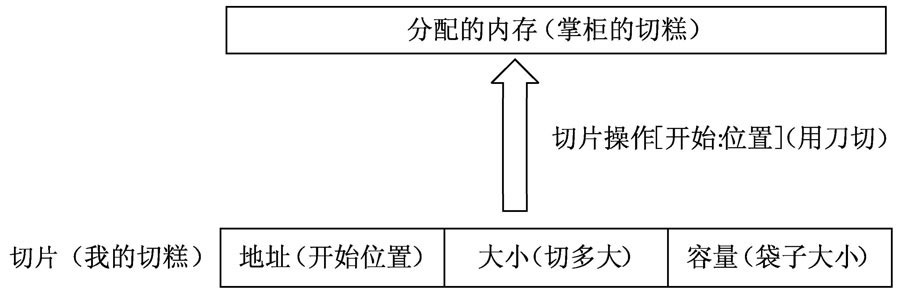2 5 数组和切片

# 2.5 数组和切片 #

## 2.5.1 Golang中的数组 #

• 数组中是固定长度的连续空间（内存区域）
• 数组中所有元素的类型是一样的
``````	var a1 int

//初始化数组
var b1 = float32{1000.0, 2.0, 3.4, 7.0, 50.0}
``````

``````//声明二维数组，只要 任意加中括号，可以声明更多维，相应占用空间指数上指
var arr int
//赋值
arr = int{
{1, 2, 3},
{2, 3, 4},
{3, 4, 5},
}
``````

## 2.5.2 何谓切片？ #

``````int* a = (int *)malloc(10);
int* b = new int(4);
``````

`Go`的数组和切片都是从`c`语言中延续过来的设计。

## 2.5.3 有何不同？ #

``````var sliceTmp []int
``````

## 2.5.4 怎么动态增加？ #

``````sliceTmp = append(sliceTmp, 4)
sliceTmp = append(sliceTmp, 5)
``````

``````for _,v := range slice1{
fmt.Println(v)
}
``````## 2.5.5 切片的长度与容量，len cap append copy #

``````slice1 := []int{1, 2, 3}
``````

``````len=3 cap=3 slice=[1 2 3]
``````

``````	slice1 = make([]int, 3, 5) // 3 是长度 5 是容量
``````

``````len=3 cap=5 slice=[0 0 0]
``````

``````slice1[len(slice1)] = 4
//报错 panic: runtime error:
//index out of range  with length 3
``````

``````slice1 = append(slice1, 4)
``````

``````len=4 cap=5 slice=[0 0 0 4]
``````

``````slice1 = append(slice1, 5)
slice1 = append(slice1, 6) // 到这里长度超过了容量，容量自动翻倍为 5*2
``````

``````len=6 cap=10 slice=[0 0 0 4 5 6]
``````

``````// 上面容量自动翻倍的过程可以看作和下面一致
slice1 = make([]int, 3, 5) // 3 是长度 5 是容量
slice1 = append(slice1, 4)
slice1 = append(slice1, 5)

// 长度不变，容量自动翻倍为 5*2
slice2 := make([]int, len(slice1),
(cap(slice1))*2)

// 拷贝 slice1 的内容到 slice2
// 注意是后面的拷贝给前面
copy(slice2, slice1)

slice2 = append(slice2, 6)
``````

## 2.5.6 切片的复制 #

``````	slice2 := make([]int, len(slice1), cap(slice1))
/* 拷贝 slice1 的内容到 slice2 */
copy(slice2, slice1) // 注意是后面的拷贝给前面
``````

``````	slice3 :=  slice2[:]
``````

``````func (b *Buffer) Bytes() []byte {
return b.buf[b.off:]
}
``````

``````	buffer := bytes.NewBuffer(make([]byte, 0, 100))
buffer.Write([]byte("abc"))
resBytes := buffer.Bytes()
fmt.Printf("%s \n", resBytes)
resBytes = 'd'
fmt.Printf("%s \n", resBytes)
fmt.Printf("%s \n", buffer.Bytes())
``````

``````abc
dbc
dbc
``````

## 2.5.7 截取部分元素 #

`slice2`的值是`[0 0 0 4 5 6]`，现在有一个需求，要截取第2个元素出来

``````slice3 := slice2[0:1]
``````

``````len=1 cap=10 slice=
``````

``````slice3 = 1
slice2 = 2
printSlice(slice2)
printSlice(slice3)
``````

``````len=6 cap=10 slice=[2 0 0 4 5 6]
len=1 cap=10 slice=
``````

``````slice2 = []int{0, 0, 0, 1, 2, 3}
slice3 = make([]int, 1, 1)
copy(slice3, slice2[0:1])
``````

## 2.5.8 工具函数补充 #

``````slice2 = []int{0, 3, 0, 1, 2, 0}
sort.Ints(slice2)
fmt.Println(slice2)
``````

``````[0 0 0 1 2 3]
``````

## 2.5.9 小结 #